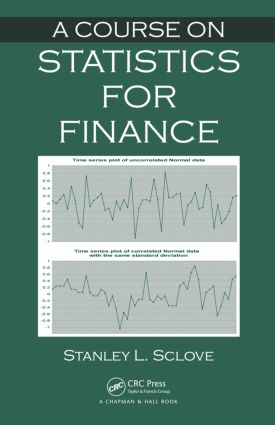# A Course on Statistics for Finance

## 1st Edition

Chapman and Hall/CRC

280 pages | 4 B/W Illus.

Hardback: 9781439892541
pub: 2012-12-06
\$115.00
x
eBook (VitalSource) : 9781315373751
pub: 2018-09-03
from \$57.50

FREE Standard Shipping!

### Description

Taking a data-driven approach, A Course on Statistics for Finance presents statistical methods for financial investment analysis. The author introduces regression analysis, time series analysis, and multivariate analysis step by step using models and methods from finance.

The book begins with a review of basic statistics, including descriptive statistics, kinds of variables, and types of data sets. It then discusses regression analysis in general terms and in terms of financial investment models, such as the capital asset pricing model and the Fama/French model. It also describes mean-variance portfolio analysis and concludes with a focus on time series analysis.

Providing the connection between elementary statistics courses and quantitative finance courses, this text helps both existing and future quants improve their data analysis skills and better understand the modeling process.

### Reviews

"… Through numerous examples, the book explains how the theory of RDS can describe the asymptotic and qualitative behavior of systems of random and stochastic differential-difference equations in terms of stability, invariant manifolds and attractors. … provides a variety of RDS for approximating financial models, and studies the stability and optimal control of RDS. The book is useful for graduate students in RDS and mathematical _nance as well as practitioners working in the financial industry."

— Ahmed Hegazi (Mansoura ), Zentralblatt MATH

INTRODUCTORY CONCEPTS AND DEFINITIONS

Review of Basic Statistics

What Is Statistics?

Characterizing Data

Measures of Central Tendency

Measures of Variability

Higher Moments

Summarizing Distributions

Bivariate Data

Three Variables

Two-Way Tables

Stock Price Series and Rates of Return

Introduction

Sharpe Ratio

Value-at-Risk

Distributions for RORs

Several Stocks and Their Rates of Return

Introduction

Review of Covariance and Correlation

Two Stocks

Three Stocks

m Stocks

REGRESSION

Simple Linear Regression; CAPM and Beta

Introduction

Simple Linear Regression

Estimation

Inference Concerning the Slope

Testing Equality of Slopes of Two Lines through the Origin

Linear Parametric Functions

Variances Dependent upon X

A Financial Application: CAPM and "Beta"

Slope and Intercept

Multiple Regression and Market Models

Multiple Regression Models

Market Models

Models with Both Numerical and Dummy Explanatory Variables

Model Building

PORTFOLIO ANALYSIS

Mean-Variance Portfolio Analysis

Introduction

Two Stocks

Three Stocks

m Stocks

m Stocks and a Risk-Free Asset

Value-at-Risk

Selling Short

Market Models and Beta

Utility-Based Portfolio Analysis

Introduction

Single-Criterion Analysis

TIME SERIES ANALYSIS

Introduction to Time Series Analysis

Introduction

Control Charts

Moving Averages

Need for Modeling

Trend, Seasonality, and Randomness

Models with Lagged Variables

Moving-Average Models

Identification of ARIMA Models

Seasonal Data

Dynamic Regression Models

Simultaneous Equations Models

Regime Switching Models

Introduction

Bull and Bear Markets

Appendix A: Vectors and Matrices

Appendix B: Normal Distributions

Appendix C: Lagrange Multipliers

Appendix D: Abbreviations and Symbols

Index

A Summary, Exercises, and Bibliography appear at the end of each chapter.

Chicago, IL, USA